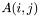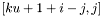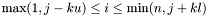# Lorene::Matrice Class Reference [Utilities.]

Matrix handling. More...

#include <matrice.h>

List of all members.

## Public Member Functions

Matrice (int size1, int size2)
Standard constructor.
Matrice (const Matrice &)
Constructor by copy.
Matrice (const Tbl &tab)
Constructor from a Tbl.
~Matrice ()
Destructor.
int get_etat () const
Returns the logical state.
void set_etat_qcq ()
Sets the logical state to ETATQCQ (ordinary state).
void set_etat_zero ()
Sets the logical state to ETATZERO (zero).
void set_etat_nondef ()
Sets the logical state to ETATNONDEF (undefined state).
void annule_hard ()
Sets the logical state to ETATQCQ (undefined state).
int get_dim (int i) const
Returns the dimension of the matrix.
Tbl get_array () const
Returns the array of matrix elements.
void operator= (double x)
Sets all the element of *std to x.
void operator= (const Matrice &)
Assignement to another Matrice.
void operator= (const Tbl &)
Assignement to a Tbl.
double & set (int j, int i)
double operator() (int j, int i) const
void set_band (int up, int low) const
Calculate the band storage of *std.
void set_lu () const
Calculate the LU-representation, assuming the band-storage has been done.
Tbl inverse (const Tbl &sec_membre) const
Solves the linear system represented by the matrix.
Tbl val_propre () const
Returns the eigenvalues of the matrix, calculated using LAPACK.
Matrice vect_propre () const
Returns the eigenvectors of the matrix, calculated using LAPACK.
double determinant () const
Computes the determinant of the matrix, using LAPACK and the standard decomposition.
Matrice transpose () const
Computes the transpose matrix.
void operator+= (const Matrice &)
Addition of a Matrice to this.
void operator+= (double)
Addition of a double to this.
void operator-= (const Matrice &)
Subtraction of a Matrice to this.
void operator-= (double)
Subtraction of a double to this.
void operator*= (double)
Multiplication of this by a double.
void operator/= (double)
Division of this by a double.

## Private Member Functions

void del_t ()
Logical destructor : dellocates the memory of the various used representations.
void del_deriv ()
Deletes the (mutable) derived members: band, lu, permute.

## Private Attributes

int etat
logical state (ETATZERO, ETATQCQ or ETATNONDEF)
Tblstd
Pointer on the array of the standard representation.
int ku
Number of upper-diagonals in the band representation.
int kl
Number of lower-diagonals in the band representation.
Tblband
Pointer on the array of the band representation of a square matrix.
Tbllu
Pointer on the first array of the LU-representation.
Itblpermute
Pointer on the second array of the LU-representation.

## Friends

ostream & operator<< (ostream &, const Matrice &)
Display.
Matrice operator+ (const Matrice &, const Matrice &)
Matrice operator- (const Matrice &, const Matrice &)
Matrice operator* (const Matrice &, double)
Matrice operator* (double, const Matrice &)
Matrice operator* (const Matrice &, const Matrice &)
Matrice operator/ (const Matrice &, double)

## Detailed Description

Matrix handling.

The matrix can be stored in the usual way in std, in a band-way by band and on a LU-decomposition by the two arrays lu and permute. All the storage conventions are those af LAPACK which is used to make the LU-decomposition, the inversion and to compute the eigenvalues of the matrix. All those representations are redondant, that means that doing the LU-decomposition, for example, does NOT destroy previously calculated type of storage.

()

Definition at line 152 of file matrice.h.

## Constructor & Destructor Documentation

 Lorene::Matrice::Matrice ( int size1, int size2 )

Standard constructor.

All the representations are set to ETATNONDEF.

Parameters:
 size1 [input] number of lines. size2 [input] number of columns.

Definition at line 206 of file matrice.C.

References band, etat, kl, ku, lu, permute, and std.

 Lorene::Matrice::Matrice ( const Matrice & source )

Constructor by copy.

Definition at line 217 of file matrice.C.

References band, etat, kl, ku, lu, permute, and std.

 Lorene::Matrice::Matrice ( const Tbl & tab )

Constructor from a Tbl.

Parameters:
 tab [input] 2-dimension or 1-dimension array

If tab is a 1-dimension Tbl, a single-column matrix is created, otherwise *std is simply constructed by a Tbl copy of tab.

Definition at line 231 of file matrice.C.

 Lorene::Matrice::~Matrice ( )

Destructor.

Definition at line 258 of file matrice.C.

References del_t().

## Member Function Documentation

 void Lorene::Matrice::annule_hard ( )

Sets the logical state to ETATQCQ (undefined state).

And sets all the components to zero

Definition at line 196 of file matrice.C.

 void Lorene::Matrice::del_deriv ( )  [private]

Deletes the (mutable) derived members: band, lu, permute.

Definition at line 167 of file matrice.C.

References band, lu, and permute.

 void Lorene::Matrice::del_t ( )  [private]

Logical destructor : dellocates the memory of the various used representations.

Definition at line 159 of file matrice.C.

References del_deriv(), and std.

 double Lorene::Matrice::determinant ( ) const

Computes the determinant of the matrix, using LAPACK and the standard decomposition.

Definition at line 563 of file matrice.C.

References get_dim(), and val_propre().

 Tbl Lorene::Matrice::get_array ( ) const [inline]

Returns the array of matrix elements.

Definition at line 251 of file matrice.h.

References std.

 int Lorene::Matrice::get_dim ( int i ) const

Returns the dimension of the matrix.

Parameters:
 i [input] if i =0 returns the number of lines and if i =2 returns the number of columns.

Definition at line 263 of file matrice.C.

References Lorene::Tbl::get_dim(), and std.

 int Lorene::Matrice::get_etat ( ) const [inline]

Returns the logical state.

Definition at line 213 of file matrice.h.

References etat.

 Tbl Lorene::Matrice::inverse ( const Tbl & sec_membre ) const

Solves the linear system represented by the matrix.

The calculus assumes the the LU-decomposition has been done and is conducted using LAPACK.

Parameters:
 sec_membre [input] the right-hand side of the system.

Definition at line 427 of file matrice.C.

 double Lorene::Matrice::operator() ( int j, int i ) const [inline]

Parameters:
 j [input] line coordinate. i [input] column coordinate.

Definition at line 290 of file matrice.h.

References Lorene::Dim_tbl::dim, Lorene::Tbl::dim, etat, std, and Lorene::Tbl::t.

 void Lorene::Matrice::operator*= ( double x )

Multiplication of this by a double.

Definition at line 625 of file matrice.C.

References std.

 void Lorene::Matrice::operator+= ( double x )

Addition of a double to this.

Definition at line 615 of file matrice.C.

References std.

 void Lorene::Matrice::operator+= ( const Matrice & a )

Addition of a Matrice to this.

Definition at line 605 of file matrice.C.

References std.

 void Lorene::Matrice::operator-= ( double x )

Subtraction of a double to this.

Definition at line 620 of file matrice.C.

References std.

 void Lorene::Matrice::operator-= ( const Matrice & a )

Subtraction of a Matrice to this.

Definition at line 610 of file matrice.C.

References std.

 void Lorene::Matrice::operator/= ( double x )

Division of this by a double.

Definition at line 630 of file matrice.C.

References std.

 void Lorene::Matrice::operator= ( const Tbl & source )

Assignement to a Tbl.

Definition at line 309 of file matrice.C.

 void Lorene::Matrice::operator= ( const Matrice & source )

Assignement to another Matrice.

Definition at line 276 of file matrice.C.

References band, del_t(), etat, Lorene::Tbl::get_dim(), kl, ku, lu, permute, set_etat_nondef(), set_etat_qcq(), set_etat_zero(), and std.

 void Lorene::Matrice::operator= ( double x )

Sets all the element of *std to x.

The other representations are set to ETATNONDEF.

Definition at line 268 of file matrice.C.

References set_etat_qcq(), set_etat_zero(), and std.

 double& Lorene::Matrice::set ( int j, int i )  [inline]

This is done in *std and all the other representations are no longer valid.

Parameters:
 j [input] line coordinate. i [input] column coordinate.

Definition at line 277 of file matrice.h.

References band, del_deriv(), Lorene::Dim_tbl::dim, Lorene::Tbl::dim, etat, lu, std, and Lorene::Tbl::t.

 void Lorene::Matrice::set_band ( int up, int low ) const

Calculate the band storage of *std.

Please note that this function does NOT check if *std represents a real band-matrix.

Parameters:
 up [input] number of upper-diagonals. low [input] number of lower-diagonals.

Definition at line 367 of file matrice.C.

References Lorene::Tbl::annule_hard(), band, Lorene::Tbl::get_dim(), kl, ku, Lorene::Tbl::set(), and std.

 void Lorene::Matrice::set_etat_nondef ( )

Sets the logical state to ETATNONDEF (undefined state).

The state of of all the representations is now ETATNONDEF.

Definition at line 190 of file matrice.C.

References del_deriv(), etat, Lorene::Tbl::set_etat_nondef(), and std.

 void Lorene::Matrice::set_etat_qcq ( )

Sets the logical state to ETATQCQ (ordinary state).

The state of *std is now ETATQCQ and the one of all the other representations is ETATNONDEF.

Definition at line 178 of file matrice.C.

References del_deriv(), etat, Lorene::Tbl::set_etat_qcq(), and std.

 void Lorene::Matrice::set_etat_zero ( )

Sets the logical state to ETATZERO (zero).

The state of *std is now ETATZERO and the one of all the other representations is ETATNONDEF.

Definition at line 184 of file matrice.C.

References del_deriv(), etat, Lorene::Tbl::set_etat_zero(), and std.

 void Lorene::Matrice::set_lu ( ) const

Calculate the LU-representation, assuming the band-storage has been done.

The calculus is done using LAPACK.

Definition at line 395 of file matrice.C.

 Matrice Lorene::Matrice::transpose ( ) const

Computes the transpose matrix.

Definition at line 581 of file matrice.C.

References etat, Lorene::Tbl::get_dim(), set(), set_etat_qcq(), set_etat_zero(), and std.

 Tbl Lorene::Matrice::val_propre ( ) const

Returns the eigenvalues of the matrix, calculated using LAPACK.

Returns:
contains the real and the imaginary parts of the eigenvalues. The real parts are in Tbl (0, *) and the imaginary parts in Tbl (1, *).

Definition at line 460 of file matrice.C.

References etat, get_dim(), Lorene::Tbl::set(), Lorene::Tbl::set_etat_qcq(), std, and Lorene::Tbl::t.

 Matrice Lorene::Matrice::vect_propre ( ) const

Returns the eigenvectors of the matrix, calculated using LAPACK.

Definition at line 510 of file matrice.C.

References etat, get_dim(), set(), set_etat_qcq(), std, and Lorene::Tbl::t.

## Friends And Related Function Documentation

 ostream& operator<< ( ostream & , const Matrice & )  [friend]

Display.

## Member Data Documentation

 Tbl* Lorene::Matrice::band [mutable, private]

Pointer on the array of the band representation of a square matrix.

To be precise,is stored in bandfor, n being the size of the matrix.

Definition at line 170 of file matrice.h.

 int Lorene::Matrice::etat [private]

logical state (ETATZERO, ETATQCQ or ETATNONDEF)

Definition at line 156 of file matrice.h.

 int Lorene::Matrice::kl [mutable, private]

Number of lower-diagonals in the band representation.

Definition at line 162 of file matrice.h.

 int Lorene::Matrice::ku [mutable, private]

Number of upper-diagonals in the band representation.

Definition at line 161 of file matrice.h.

 Tbl* Lorene::Matrice::lu [mutable, private]

Pointer on the first array of the LU-representation.

Definition at line 173 of file matrice.h.

 Itbl* Lorene::Matrice::permute [mutable, private]

Pointer on the second array of the LU-representation.

Definition at line 174 of file matrice.h.

 Tbl* Lorene::Matrice::std [private]

Pointer on the array of the standard representation.

Definition at line 158 of file matrice.h.

The documentation for this class was generated from the following files:

Generated on 7 Dec 2019 for LORENE by1.6.1